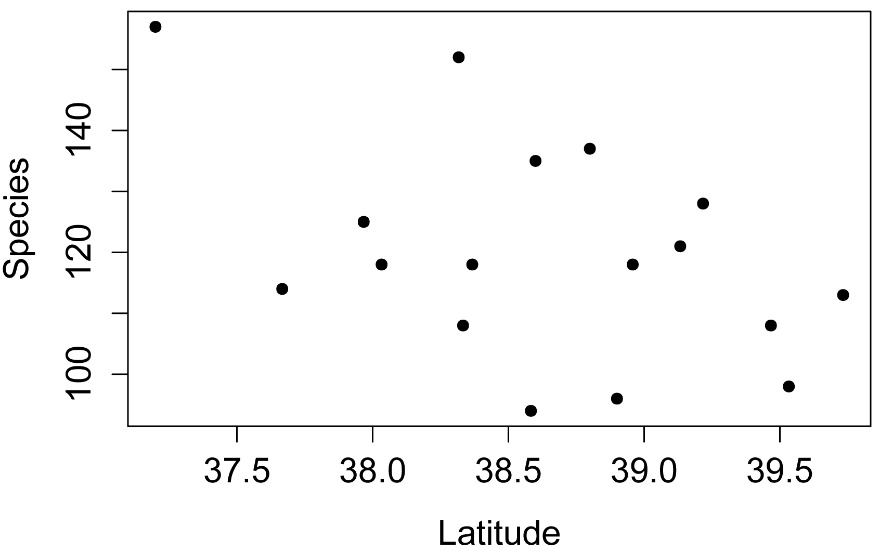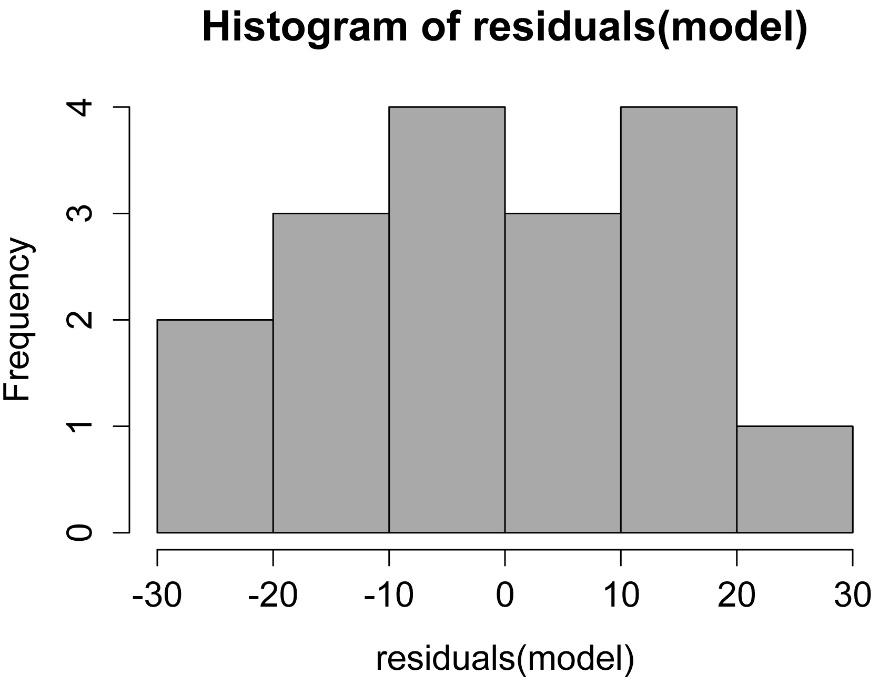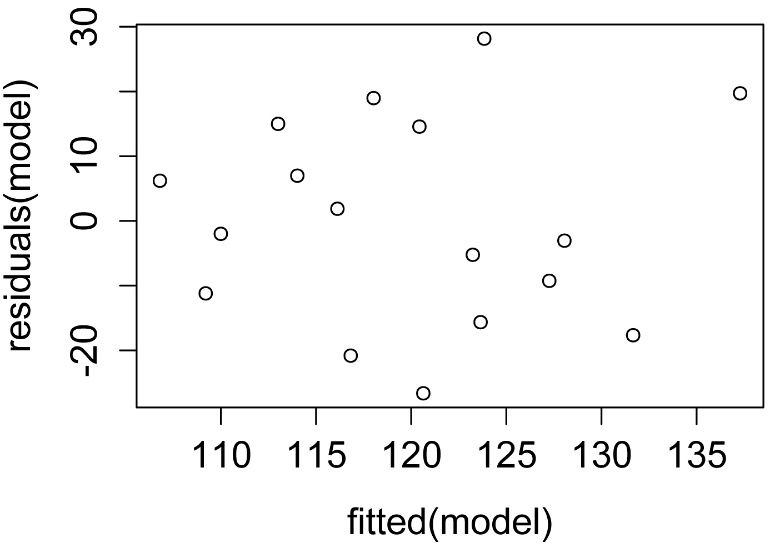## An R Companion for the Handbook of Biological Statistics

Salvatore S. Mangiafico

# Correlation and Linear Regression

Introduction

The amphipod egg example is shown below in the “How to do the test” section.

When to use them

Correlation versus linear regression

Correlation and causation

Null hypothesis

Independent vs. dependent variables

How the test works

Assumptions

See the Handbook for information on these topics.

Examples

The species diversity example is shown below in the “How to do the test” section.

Graphing the results

Similar tests

### How to do the test

#### Correlation and linear regression example

### --------------------------------------------------------------
### Correlation and linear regression, species diversity example
### pp. 207–208
### --------------------------------------------------------------

Input = ("
Town                  State  Latitude  Species
'Bombay Hook'          DE     39.217    128
'Cape Henlopen'        DE     38.800    137
'Middletown'           DE     39.467    108
'Milford'              DE     38.958    118
'Rehoboth'             DE     38.600    135
'Seaford-Nanticoke'    DE     38.583     94
'Wilmington'           DE     39.733    113
'Crisfield'            MD     38.033    118
'Denton'               MD     38.900     96
'Elkton'               MD     39.533     98
'Lower Kent County'    MD     39.133    121
'Ocean City'           MD     38.317    152
'Salisbury'            MD     38.333    108
'S Dorchester County'  MD     38.367    118
'Cape Charles'         VA     37.200    157
'Chincoteague'         VA     37.967    125
'Wachapreague'         VA     37.667    114
")

##### Simple plot of the data

plot(Species ~ Latitude,
data=Data,
pch=16,
xlab = "Latitude",
ylab = "Species")#### Correlation

Correlation can be performed with the cor.test function in the native stats package.  It can perform Pearson, Kendall, and Spearman correlation procedures.  Methods for multiple correlation of several variables simultaneously are discussed in the Multiple regression chapter.

#### Pearson correlation

Pearson correlation is the most common form of correlation.  It is a parametric test, and assumes that the data are linearly related and that the residuals are normally distributed.

cor.test( ~ Species + Latitude,
data=Data,
method = "pearson",
conf.level = 0.95)

Pearson's product-moment correlation

t = -2.0225, df = 15, p-value = 0.06134

cor

-0.4628844

#### Kendall correlation

Kendall rank correlation is a non-parametric test that does not assume a distribution of the data or that the data are linearly related.  It ranks the data to determine the degree of correlation.

cor.test( ~ Species + Latitude,
data=Data,
method = "kendall",
continuity = FALSE,
conf.level = 0.95)

Kendall's rank correlation tau

z = -1.3234, p-value = 0.1857

tau

-0.2388326

#### Spearman correlation

Spearman rank correlation is a non-parametric test that does not assume a distribution of the data or that the data are linearly related.  It ranks the data to determine the degree of correlation, and is appropriate for ordinal measurements.

cor.test( ~ Species + Latitude,
data=Data,
method = "spearman",
continuity = FALSE,
conf.level = 0.95)

Spearman's rank correlation rho

S = 1111.908, p-value = 0.1526

rho

-0.3626323

#### Linear regression

Linear regression can be performed with the lm function in the native stats package.  A robust regression can be performed with the lmrob function in the robustbase package.

model = lm(Species ~ Latitude,
data = Data)

summary(model)                    # shows parameter estimates,
# p-value for model, r-square

Estimate Std. Error t value Pr(>|t|)

(Intercept)  585.145    230.024   2.544   0.0225 *

Latitude     -12.039      5.953  -2.022   0.0613 .

Multiple R-squared:  0.2143,  Adjusted R-squared:  0.1619

F-statistic:  4.09 on 1 and 15 DF,  p-value: 0.06134

library(car)

Anova(model, type="II")              # shows p-value for effects in model

Response: Species

Sum Sq Df F value  Pr(>F)

Latitude  1096.6  1  4.0903 0.06134 .

Residuals 4021.4 15

##### Plot linear regression

int =  model\$coefficient["(Intercept)"]
slope =model\$coefficient["Latitude"]

plot(Species ~ Latitude,
data = Data,
pch=16,
xlab = "Latitude",
ylab = "Species")

abline(int, slope,
lty=1, lwd=2, col="blue")     #  style and color of line##### Checking assumptions of the model

hist(residuals(model),
col="darkgray")A histogram of residuals from a linear model.  The distribution of these residuals should be approximately normal.

plot(fitted(model),
residuals(model))A plot of residuals vs. predicted values.  The residuals should be unbiased and homoscedastic.  For an illustration of these properties, see this diagram by Steve Jost at DePaul University: condor.depaul.edu/sjost/it223/documents/resid-plots.gif.

### additional model checking plots with: plot(model)
### alternative: library(FSA); residPlot(model)

#### Robust regression

The lmrob function in the robustbase package produces a linear regression which is not sensitive to outliers in the response variable.  It uses MM-estimation.

library(robustbase)

model = lmrob(Species ~ Latitude,
data = Data)

summary(model)                    # shows parameter estimates, r-square

Estimate Std. Error t value Pr(>|t|)

(Intercept)  568.830    230.203   2.471   0.0259 *

Latitude     -11.619      5.912  -1.966   0.0681 .

Multiple R-squared:  0.1846,  Adjusted R-squared:  0.1302

model.null = lmrob(Species ~ 1,
data = Data)

anova(model, model.null)         # shows p-value for model

pseudoDf Test.Stat Df Pr(>chisq)

1       15

2       16    3.8634  1    0.04935 *

##### Plot the model

int =  model\$coefficient["(Intercept)"]
slope =model\$coefficient["Latitude"]

plot(Species ~ Latitude,
data = Data,
pch=16,
xlab = "Latitude",
ylab = "Species")

abline(int, slope,
lty=1, lwd=2, col="blue")      #  style and color of line#     #     #

#### Linear regression example

### --------------------------------------------------------------
### Linear regression, amphipod eggs example
### pp. 191–193
### --------------------------------------------------------------

Input = ("
Weight  Eggs
5.38    29
7.36    23
6.13    22
4.75    20
8.10    25
8.62    25
6.30    17
7.44    24
7.26    20
7.17    27
7.78    24
6.23    21
5.42    22
7.87    22
5.25    23
7.37    35
8.01    27
4.92    23
7.03    25
6.45    24
5.06    19
6.72    21
7.00    20
9.39    33
6.49    17
6.34    21
6.16    25
5.74    22
")

model = lm(Eggs ~ Weight,
data = Data)

summary(model)                    # shows parameter estimates,
# p-value for model, r-square

Coefficients:

Estimate Std. Error t value Pr(>|t|)

(Intercept)  12.6890     4.2009   3.021   0.0056 **

Weight        1.6017     0.6176   2.593   0.0154 *

Multiple R-squared:  0.2055,  Adjusted R-squared:  0.175

F-statistic: 6.726 on 1 and 26 DF,  p-value: 0.0154

###  Neither the r-squared nor the p-value agrees with what is reported

###    in the Handbook.

library(car)

Anova(model, type="II")           # shows p-value for effects in model

Sum Sq Df F value Pr(>F)

Weight     93.89  1  6.7258 0.0154 *

Residuals 362.96 26

#     #     #

### Power analysis

#### Power analysis for correlation

### --------------------------------------------------------------
### Power analysis, correlation, p. 208
### --------------------------------------------------------------

pwr.r.test(n = NULL,
r = 0.500,
sig.level = 0.05,
power = 0.80,
alternative = "two.sided")

approximate correlation power calculation (arctangh transformation)

n = 28.87376   # answer is somewhat different than in Handbook

#     #     #